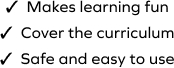# Identify the Sum of a 2-digit Number and Tens

SplashLearn - The complete PreK - Grade 5 Math & ELA Learning Program Built for Your Child
Home > Identify the Sum of a 2-digit Number and Tens
The game requires students to work with a set of problems on addition and use their conceptual understanding to add multiples of 10 to a 2-digit number. The student will identify the sum of a 2-digit number and tens in this game. Students will select the correct option to complete the addition equation.